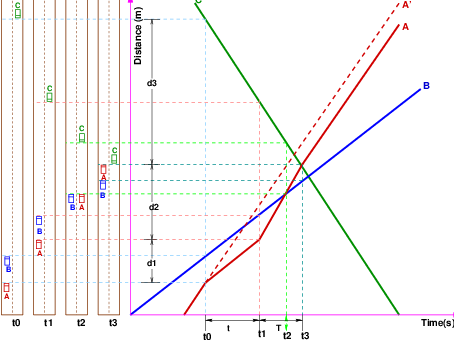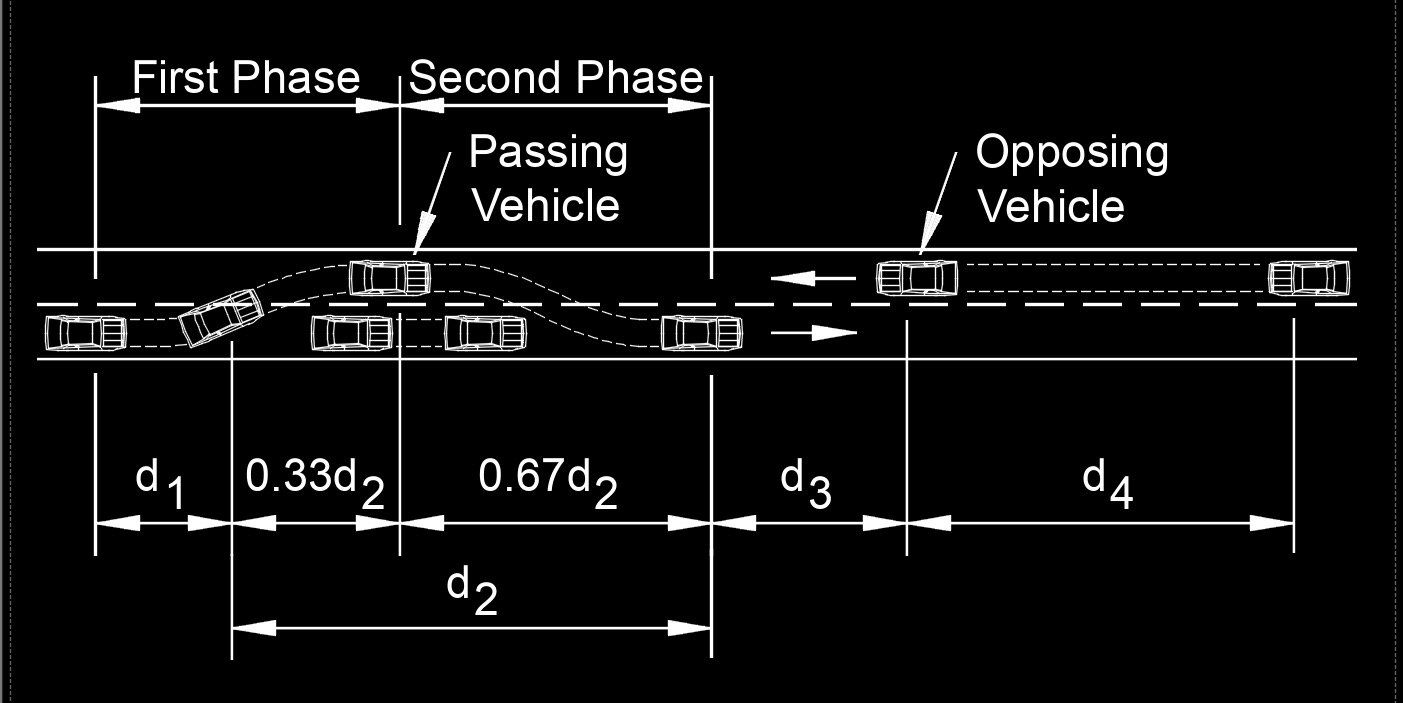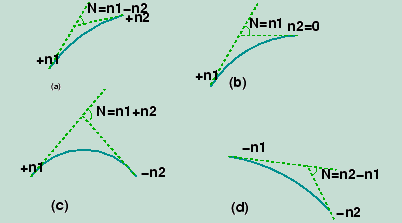# Geometric Design III

Overtaking Sight Distance

The overtaking sight distance is the minimum distance open to the vision of the driver of a vehicle intending to overtake the slow vehicle ahead safely against the traffic in the opposite direction. The overtaking sight distance or passing sight distance is measured along the centerline of the road over which a driver with his eye level 1.2 m above the road surface can see the top of an object 1.2 m above the road surface. The factors that affect the OSD are:

• Velocities of the overtaking vehicle, overtaken vehicle and of the vehicle coming in the opposite direction.

• Spacing between vehicles, which in-turn depends on the speed

• Skill and reaction time of the driver

• Rate of acceleration of overtaking vehicleTime-space diagram: Illustration of overtaking sight distance

The dynamics of the overtaking operation is given in the figure which is a time-space diagram. The x-axis denotes the time and the y-axis shows the distance traveled by the vehicles. The trajectory of the slow moving vehicle (B) is shown as a straight line which indicates that it is traveling at a constant speed. A fast moving vehicle (A) is traveling behind vehicle B.

The trajectory of the vehicle is shown initially with a steeper slope. The dotted line indicates the path of the vehicle A if B was absent. The vehicle A slows down to follow the vehicle B as shown in the figure with the same slope from t0 to t1. Then it overtakes vehicle B and occupies the left lane at time t3. The time duration T = t3 − t1 is the actual duration of the overtaking operation. The snapshots of the road at time t0, t1, and t3 are shown on the left side of the figure.

From the overtaking sight distance consists of three parts. d1 the distance traveled by overtaking vehicle A during the reaction time t = t1 − t0 d2 the distance traveled by the vehicle during the actual overtaking operation T = t3 − t1 d3 is the distance traveled by on-coming vehicle C during the overtaking operation (T).

THEREFORE OSD=d1+d2+d3

It is assumed that the vehicle A is forced to reduce its speed to vb, the speed of the slow moving vehicle B and travels behind it during the reaction time t of the driver. So d1 is given by:

d1=vbt

Then vehicle A starts to accelerate, shifts the lane, overtake and shifts back to the original lane. The vehicle A maintains the spacing s before and after overtaking. The spacing s in m is given by:

s=0.7vb + 6

Let T be the duration of actual overtaking. The distance traveled by B during the overtaking operation is 2s+vbT. Also, during this time, vehicle A accelerated from initial velocity vb and overtaking is completed while reaching final velocity v. Hence the distance traveled is given by:The distance traveled by the vehicle C moving at design speed v m/sec during overtaking operation is given by: d3 = vT

Where vb is the velocity of the slow moving vehicle in m/sec2 , the reaction time of the driver in sec, s is the spacing between the two vehicles in m given by equation 13.5 and a is the overtaking vehicle's acceleration in m/sec2 .

In case the speed of the overtaken vehicle is not given, it can be assumed that it moves 16 kmph slower than the design speed. On divided highways, d3 need not be considered.

On divided highways with four or more lanes, IRC suggests that it is not necessary to provide the OSD, but only SSD is sufficient.

QUESTION-

At highway stretches where the required overtaking sight distance cannot be provided, it is necessary to incorporate :

1. At least twice the SSD

2. Half the required OSD

3. One-third of OSD

4. Three times the SSD

 At highway stretches where the required overtaking sight distance cannot be provided, it is necessary to incorporate : At least twice the SSD Half the required OSD One-third of OSD Three times the SSD ANSWER At least twice the SSD   QUESTION   A super-elevation e is provided on a circular horizontal curve such that a vehicle can be stopped on the curve without sliding. Assuming a design speed v and maximum coefficient of side friction fmax, which one of the following criteria should be satisfied ?   ANSWER (e less than equal to fmax    QUESTION  i) List and draw the various vertical curves adopted in highways.  (ii) Explain the controls and guidelines for safe, comfortable travel in highway vertical curves.  (iii) List the various technical guidelines recommended for safety and comfort in case of horizontal curves in highway ANSWER SUMMIT CURVE  Summit curves are vertical curves with gradients upwards. They are formed when two gradients meet as illustrated in figure 1 in any of the following four ways:  1. When a positive gradient meets another positive gradient [figure 1a]  2. When a positive gradient meets a flat gradient [figure 1b].  3. When an ascending gradient meets a descending gradient [figure 1c]  4. When a descending gradient meets another descending gradient [figure 1d]  Type of Summit Curve Many curve forms can be used with satisfactory results, the common practice has been to use parabolic curves in summit curves. This is primarily because of the ease with which it can be laid out as well as allowing a comfortable transition from one gradient to another. Although a circular curve offers equal sight distance at every point on the curve, for very small deviation angles a circular curve and parabolic curves are almost congruent. Furthermore, the use of parabolic curves were found to give excellent riding comfort.DESIGN CONSIDERATION In determining the type and length of the vertical curve, the design considerations are comfort and security of the driver, and the appearance of the profile alignment. Among these, sight distance requirements for the safety are most important on summit curves.  The stopping sight distance or absolute minimum sight distance should be provided on these curves and where overtaking is not prohibited, overtaking sight distance or intermediate sight distance should be provided as far as possible.  When a fast moving vehicle travels along a summit curve, there is less discomfort to the passengers. This is because the centrifugal force will be acting upwards while the vehicle negotiates a summit curve which is against the gravity and hence a part of the tyre pressure is relieved.  Also if the curve is provided with adequate sight distance, the length would be sufficient to ease the shock due to change in gradient.   Circular summit curves are identical since the radius remains same throughout and hence the sight distance. From this point of view, transition curves are not desirable since they have varying radius and so the sight distance will also vary.  The deviation angles provided on summit curves for highways are very large, and so the simple parabola is almost congruent to a circular arc, between the same tangent points. Parabolic curves are easy for computation and also it has been found out that it provides good riding comfort to the drivers. It is also easy for field implementation. Due to all these reasons, a simple parabolic curve is preferred as a summit curve. QUESTION Explain in brief the various classifications of rural roads with its salient components as per IRC Standards with neat sketches. The Indian Highways as classified in types of roads are as follows. (i) National Highways (NH) (ii) State Highways (SH) (iii) Major District Roads (MDR) (iv) Other District Highways (ODR) (v) Village Roads (VR)